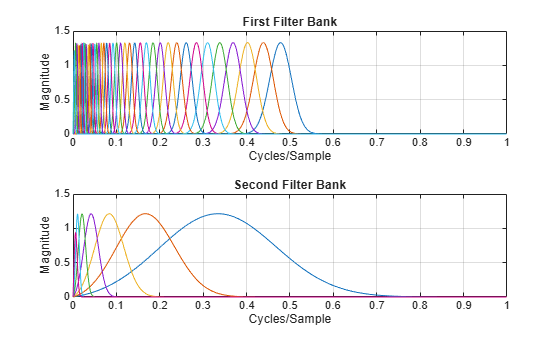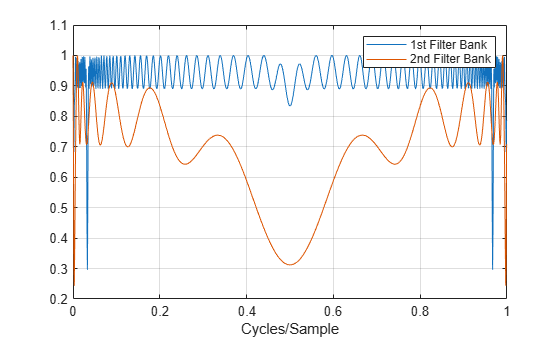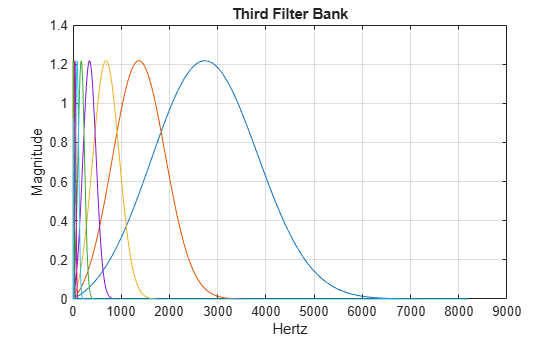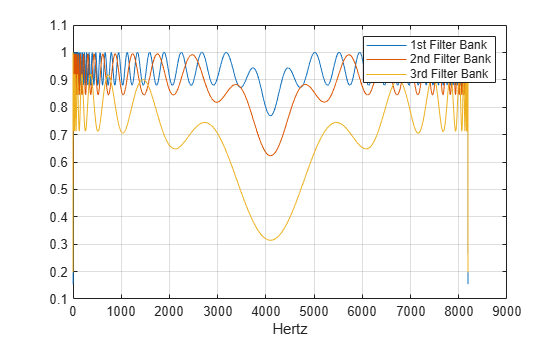Main Content

# waveletScattering

Wavelet time scattering

## Description

Use the `waveletScattering` object to create a network for a wavelet time scattering decomposition using the Gabor (analytic Morlet) wavelet. The network uses wavelets and a lowpass scaling function to generate low-variance representations of real-valued time series data. Wavelet time scattering yields representations insensitive to translations in the input signal without sacrificing class discriminability. You can use the representations as inputs to a classifier. You can specify the duration of translation invariance and the number of wavelet filters per octave. The scattering network also supports time × channel × batch (T×C×B) inputs.

## Creation

### Syntax

``sf = waveletScattering``
``sf = waveletScattering(Name,Value)``

### Description

example

````sf = waveletScattering` creates a wavelet time scattering network with two filter banks. The first filter bank has a quality factor of eight wavelets per octave. The second filter bank has a quality factor of one wavelet per octave. By default, `waveletScattering` assumes a signal input length of 1024 samples. The scale invariance length is 512 samples. By default, `waveletScattering` uses periodic boundary conditions.```

example

````sf = waveletScattering(Name,Value)` creates a network for wavelet scattering, `sf`, with properties specified by one or more `Name,Value` pair arguments. Properties can be specified in any order as `Name1,Value1,...,NameN,ValueN`. Enclose each property name in quotes. NoteWith the exception of `OversamplingFactor`, after creation you cannot change a property value of an existing scattering network. For example, if you have a network `sf` with a `SignalLength` of 2000, you must create a second network `sf2` for a signal with 2001 samples. You cannot assign a different `SignalLength` to `sf`. ```

## Properties

expand all

Signal length in samples, specified as a positive integer ≥ 16. If the input to the scattering network is a row vector, `SignalLength` must match the number of columns in the input data. If the input to the scattering network is a column vector, matrix, or 3-D array, `SignalLength` must match the number of rows in the data.

Data Types: `double`

Sampling frequency in hertz, specified as a positive scalar. If unspecified, frequencies are in cycles/sample and the Nyquist frequency is ½.

Data Types: `double`

Scattering transform invariance scale, specified as a positive scalar. `InvarianceScale` specifies the translation invariance of the scattering transform. If you do not specify `SamplingFrequency`, `InvarianceScale` is measured in samples. If you specify `SamplingFrequency`, `InvarianceScale` is measured in seconds. By default, `InvarianceScale` is one-half the `SignalLength` in samples.

`InvarianceScale` cannot exceed `SignalLength` in samples.

Example: ```sf = waveletScattering('SignalLength',1000,'SamplingFrequency',200,'InvarianceScale',5)``` has the largest possible `InvarianceScale`.

Data Types: `double`

Scattering filter bank Q factors, specified as a positive integer or a vector of positive integers. A filter bank Q factor is the number of wavelet filters per octave. Quality factors cannot exceed 32 and must be greater than or equal to 1.

If `QualityFactors` is specified as a vector, the elements of `QualityFactors` must be strictly decreasing.

Example: `sf = waveletScattering('QualityFactors',[8 2 1])` creates a wavelet scattering network with three filter banks.

Data Types: `double`

Signal extension method to apply at the boundary:

• `'periodic'` — Extend signal periodically to length `2^ceil(log2(N))`, where `N` is the signal length.

• `'reflection'` — Extend signal by reflection to length `2^ceil(log2(2 N))`, where `N` is the signal length.

The signal is extended to match the length of the wavelet filters. The length of the filters are powers of two.

The signal extension method is for internal operations. Results are downsampled back onto the scale of the original signal before being returned.

Precision of scattering decomposition:

• `'double'` — Double precision

• `'single'` — Single precision

All calculations involving the wavelet scattering network are carried out in `Precision`. Wavelet scattering functions such as `featureMatrix` and `filterbank` return outputs such as filters in `Precision`. The precision of the output of the `scatteringTransform` function does not exceed the precision of `sf`.

Note

• If you construct a scattering network with double-precision filters and apply the network to single-precision data, the filters are cast internally to single-precision. Subsequent filtering is done with single precision until a new network is created regardless of input data type.

• Specifying `Precision` as `'single'` at construction is especially useful in code generation because it eliminates the need to create an extra copy of the scattering filter banks.

Oversampling factor, specified as a nonnegative integer or `Inf`. The factor specifies how much the scattering coefficients are oversampled with respect to the critically downsampled values. The factor is on a log2 scale. By default, `OversamplingFactor` is set to `0`, which corresponds to critically downsampling the coefficients. You can use `numCoefficients` to determine the number of coefficients obtained for a scattering network. To obtain a fully undecimated scattering transform, set `OversamplingFactor` to `Inf`.

Setting `OversamplingFactor` to a value that would result in more coefficients than samples is equivalent to setting `OversamplingFactor` to `Inf`. Increasing the `OversamplingFactor` significantly increases the computational complexity and memory requirements of the scattering transform.

Example: If `sf = waveletScattering('OversamplingFactor',2)`, the scattering transform returns `22` times as many coefficients for each scattering path with respect to the critically sampled number.

Optimize scattering transform logical which determines whether the scattering transform reduces the number of scattering paths to compute based on a bandwidth consideration, specified as a numeric or logical `1` (`true`) or `0` (`false`).

If you specify `OptimizePath` as `true`, the scattering transform excludes scattering paths of order 2 and greater which do not satisfy the following criterion: The center frequency minus ½ the 3-dB bandwidth of the wavelet filter in the (i+1)th filter bank must overlap 0 (DC) plus ½ the 3-dB bandwidth of the wavelet filter in the ith filter bank. If this criterion is not satisfied, the higher-order path is excluded. Setting `OptimizePath` to true can significantly reduce the number of scattering paths and computational complexity of the scattering transform for most networks.

You can use `paths` to determine which and how many scattering paths are computed.

## Object Functions

 `scatteringTransform` Wavelet 1-D scattering transform `featureMatrix` Scattering feature matrix `log` Natural logarithm of scattering transform `filterbank` Wavelet time scattering filter banks `littlewoodPaleySum` Littlewood-Paley sum `scattergram` Visualize scattering or scalogram coefficients `centerFrequencies` Wavelet scattering bandpass center frequencies `numorders` Number of scattering orders `numfilterbanks` Number of scattering filter banks `numCoefficients` Number of wavelet scattering coefficients `paths` Scattering network paths

## Examples

collapse all

Create a wavelet time scattering network with default values.

`sf = waveletScattering`
```sf = waveletScattering with properties: SignalLength: 1024 InvarianceScale: 512 QualityFactors: [8 1] Boundary: 'periodic' SamplingFrequency: 1 Precision: 'double' OversamplingFactor: 0 OptimizePath: 0 ```

Plot the wavelet filters used in the first and second filter banks.

```[filters,f] = filterbank(sf); plot(f,filters{2}.psift) title('First Filter Bank') xlabel('Cycles/Sample') ylabel('Magnitude') grid on``````figure plot(f,filters{3}.psift) title('Second Filter Bank') xlabel('Cycles/Sample') ylabel('Magnitude') grid on```Plot the Littlewood-Paley sums of the filter banks.

```[lpsum,f] = littlewoodPaleySum(sf); figure plot(f,lpsum) legend('1st Filter Bank','2nd Filter Bank') xlabel('Cycles/Sample') grid on```This example shows how to create and apply a wavelet time scattering network with three filter banks to data.

Load in a data set. Create a scattering network with three filter banks that can be applied to the data.

```load handel disp(['Data Sampling Frequency: ',num2str(Fs),' Hz'])```
```Data Sampling Frequency: 8192 Hz ```
```sf = waveletScattering('SignalLength',numel(y),... 'SamplingFrequency',Fs,... 'QualityFactors',[4 2 1])```
```sf = waveletScattering with properties: SignalLength: 73113 InvarianceScale: 4.4625 QualityFactors: [4 2 1] Boundary: 'periodic' SamplingFrequency: 8192 Precision: 'double' OversamplingFactor: 0 OptimizePath: 0 ```

Inspect the network. Plot the wavelet filters used in the third filter bank.

```[filters,f] = filterbank(sf); plot(f,filters{4}.psift) title('Third Filter Bank') xlabel('Hertz') ylabel('Magnitude') grid on```Plot the Littlewood-Paley sums of the three filter banks.

```[lpsum,f] = littlewoodPaleySum(sf); figure plot(f,lpsum) xlabel('Hertz') grid on legend('1st Filter Bank','2nd Filter Bank','3rd Filter Bank')```Calculate the wavelet 1-D scattering transform of the data for `sf`. Visualize the scattergram of the scalogram coefficients for the first filter bank.

```[S,U] = scatteringTransform(sf,y); figure scattergram(sf,U,'FilterBank',1)```## Compatibility Considerations

expand all

Errors starting in R2021a

Behavior changed in R2021a

 Andén, Joakim, and Stéphane Mallat. “Deep Scattering Spectrum.” IEEE Transactions on Signal Processing 62, no. 16 (August 2014): 4114–28. https://doi.org/10.1109/TSP.2014.2326991.

 Mallat, Stéphane. “Group Invariant Scattering.” Communications on Pure and Applied Mathematics 65, no. 10 (October 2012): 1331–98. https://doi.org/10.1002/cpa.21413.

## SupportGet trial now